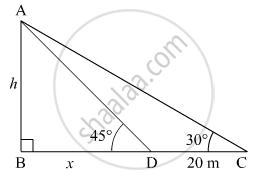# An Observer Finds the Angle of Elevation of the Top of the Tower from a Certain Point on the Ground as 30°. If the Observe Moves 20 M Towards the Base of the Tower, the Angle of Elevation of the Top Increases by 15°, Find the Height of the Tower. - Mathematics

An observer finds the angle of elevation of the top of the tower from a certain point on the ground as 30°. If the observe moves 20 m towards the base of the tower, the angle of elevation of the top increases by 15°, find the height of the tower.

#### Solution

Let the observer be at point C on the ground.

∴ ∠C = 30°

He moves 20 m towards the tower and reaches point D.

∴ ∠D = 30° + 15° = 45°

Let the distance BD be x m and the height of the tower be h m.In ΔABD

tan 45^@ = "AB"/"BD" = h/x

=> 1 = h/x

=> h = x

In ΔABC

tan 30^@ = (AB)/(BC) = h/(x + 20) = h/(h + 20)

=> 1/sqrt3 = h/(h + 20)

=> h + 20 = sqrt3h

=> h = 20/(sqrt3 - 1) =10(sqrt3 + 1) m

Hence, the height of the tower is 10(sqrt3+1) m

Concept: Heights and Distances
Is there an error in this question or solution?